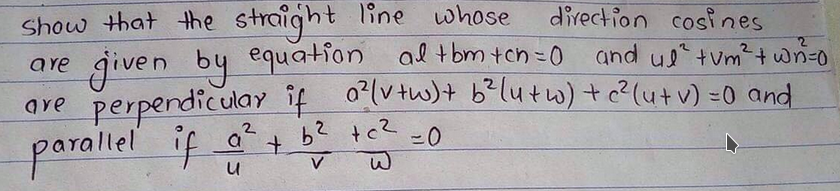Click to Chat

1800-1023-196

+91-120-4616500

CART 0

• 0

MY CART (5)

Use Coupon: CART20 and get 20% off on all online Study Material

ITEM
DETAILS
MRP
DISCOUNT
FINAL PRICE
Total Price: Rs.

There are no items in this cart.
Continue Shopping
`        reply to the question shown in the image …...............................`
one year ago

```							hello abhishek. this is a really easy ques.let the direction cosines be (l,m,n). or direction ratios are equivalently (1,m/l, n/l).let m/l=k and n/l=pso we are given a+bk+cp=0 and u+vk^2+wp^2=0these are two equations in two variables k and p. eliminate k to obtain a quadratic eqn in just p. similarly eliminate p to obtain a quadratic eqn in just k.now if the two lines are perpendicular then (1,k1,p1).(1,k2,p2)=0, where (.) is the dot product.or 1+k1k2+p1p2=0since we have a quad eqn in k, we can easily find the product of its roots k1k2 and similarly for p1p2.plug in those values and there you have it!for the parallel case, obviously (1,k1,p1)= λ(1,k2,p2)1=λ, k1=λk2 and p1=λp2or k1=k2 and p1=p2now, for the roots of any quad eqn Ax^2+Bx+C=0 to be equal, we have B^2=4AC.apply this above condition and you will obtain the necessary result!
```
one year ago
Think You Can Provide A Better Answer ?

## Other Related Questions on Magical Mathematics[Interesting Approach]

View all Questions »### Course Features

• 731 Video Lectures
• Revision Notes
• Previous Year Papers
• Mind Map
• Study Planner
• NCERT Solutions
• Discussion Forum
• Test paper with Video Solution### Course Features

• 731 Video Lectures
• Revision Notes
• Test paper with Video Solution
• Mind Map
• Study Planner
• NCERT Solutions
• Discussion Forum
• Previous Year Exam Questions